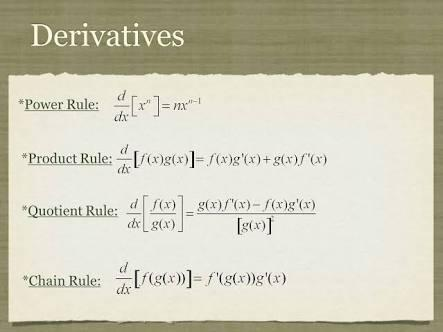# Product, Quotient & Chain Rules Assessment Test

10 Questions | Total Attempts: 146SettingsIn calculus, the product, quotient, and chain rules are methods of finding the derivative of a function that is the ratio of two differentiable functions, differentiating problems where one function is multiplied by another, and differentiating compositions of functions.

• 1.
If the two functions f(x) and g(x) are differentiable (i.e. the derivative exist) then the product is differentiable is called the...
• A.

Product rule

• B.

Quotient rule

• C.

Power rule

• D.

Chain rule

• 2.
A method of finding the derivative of a function that is the ratio of two differentiable functions is...
• A.

Quotient rule

• B.

Chain rule

• C.

Power rule

• D.

Product rule

• 3.
What can be used to find the derivative of a function?
• A.

Quotient rule

• B.

Calculator

• C.

Angle

• D.

Bodmas

• 4.
The answer after you divide one number by another is the...
• A.

Abstract

• B.

Product

• C.

Quotient

• D.

Rule

• 5.
The product of two or more numbers raised to a power is equal to the product of each number raised to the same power is...
• A.

Product rule

• B.

Power rule

• C.

Chain rule

• D.

Quotient rule

• 6.
The number which we divide is called the...
• A.

Dividend

• B.

Divisor

• C.

Quotient

• D.

• 7.
The number by which we divide is called the...
• A.

• B.

Dividend

• C.

Divident

• D.

Divisor

• 8.
The number left over after division is called the...
• A.

Dividor

• B.

Product

• C.

Dividend

• D.

Remainder

• 9.
To divide two exponents with the same base, keep the base and subtract the powers. This is...
• A.

Quotient rule

• B.

Power rule

• C.

Chain rule

• D.

Power rule

• 10.
The quantity produced by the division of two numbers is...
• A.

Quotient

• B.

Product

• C.

Multiplier

• D.

Ratio

Related TopicsBack to top